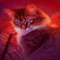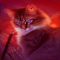# One Pixel Adversarial Attack - RCTF catspy

This post is about turning a photo of a cat into a photo of a goldfish by changing only one pixel, at least according to resnet50. With Organizers we participated in RCTF during the close race at the end 2022 to be #1 on CTFtime. This literally meant to participate in every high rated CTF and solving every challenge, including the miscy of the misc. The challenge catspy appeared at around 2am in the misc category and the description states:

This is a world where there are only cats and dogs and they are at war with each other. You, as a cat spy, want to infiltrate into the dog camp. But the dogs have installed a simple cat identifier and you can only change one pixel to avoid being identified.

Can you attack the image in `/static/start.png`? you can only change one pixel! The image format must be 60 x 60. If you upload a 60 x 60 image that only change one pixel, the system will return you a result.

Here is `start.png`:This challenge started as a black-box adversarial machine learning challenge. Given an image of a cat, we are allowed to change one pixel in the picture, so that the model does not recognize that there is a cat in the image anymore. The website only outputted the current prediction and the probability of that prediction.

However, after just a few minutes the challenge was taken offline again, because the server couldn’t stand the load. No one could have expected a black-box optimization challenge to cause a lot of load. After a random time, the challenge was released again, which is unfortunate in combination with the first blood bonus. This time source code was given.

``````def checkImg(Img):
im = Image.open('static/start.png').convert('RGB')
Img = Img.convert('RGB')
if Img.size != (60, 60):
return 0
count = 0
for i in range(60):
for j in range(60):
if im.getpixel((i,j)) != Img.getpixel((i,j)):
count += 1
if count == 1:
return 1
else:
return 0

def divide(img):
# Step 1: Initialize model with the best available weights
weights = ResNet50_Weights.DEFAULT
model = resnet50(weights=weights)
model.eval()

# Step 2: Initialize the inference transforms
preprocess = weights.transforms()

# Step 3: Apply inference preprocessing transforms
batch = preprocess(img).unsqueeze(0)

# Step 4: Use the model and print the predicted category
prediction = model(batch).squeeze(0).softmax(0)
class_id = prediction.argmax().item()
score = prediction[class_id].item()
category_name = weights.meta["categories"][class_id]
return category_name,score

@app.route('/', methods=['POST', 'GET'])
def welcome():
return render_template("index.html")

if request.method == 'POST':
f = request.files['file']
im = Image.open(f)
if checkImg(im) == 0:
return render_template('upload.html', error="image format error! the image size must be 60 x 60 and you can only change o
ne pixel!")
category_name,score = divide(im)
if category_name == 'tabby' or "cat" in category_name:
return render_template('upload.html', res=category_name + "  " + str(score))
else:
if __name__ == '__main__':
app.run(host='0.0.0.0', port=5000)
``````

The interesting information is that we have the exact model, a pretrained resnet50 and that the input image is scaled to fit the input.

While the challenge was online the first time, I started implementing https://arxiv.org/abs/1710.08864 using differential evolution. When the source model was released, I was about to implement a gradient descent based technique, which is complicated by the fact that the image is scaled, but as one does, I also wrote a brute force script to run in the background. The brute force script sets every pixel to light yellow and keeps the three positions with the minimal probability for the cat classes. Shortly after I saw that, (31, 8) had a pretty low confidence for the cat classes. Therefore, I set this pixel to random colors I did this in the hope of getting a good initialization for one of the other approaches.

``````import cv2
from torchvision.models import resnet50, ResNet50_Weights
from PIL import Image
import random

weights = ResNet50_Weights.DEFAULT
model = resnet50(weights=weights)
model.eval()
preprocess = weights.transforms()
mins = [1, 1, 1]
for _ in range(200):
rand = [random.randint(0, 255) for _ in range(3)]
image[8, 31] = rand
# very perf!1! (it is 4am leave me alone)
cv2.imwrite("tmp.png", image)
im = Image.open("tmp.png")
x = preprocess(im).unsqueeze(0)
prediction = model(x).squeeze(0).softmax(0)
class_id = prediction.argmax().item()
score = prediction[class_id].item()
category_name = weights.meta["categories"][class_id]
if (category_name == 'tabby' or "cat" in category_name):
if score < max(mins):
mins = score
mins.sort()
print(score, category_name, rand)
else:
print(score,  category_name, rand)
im.save("flag.png")
exit(0)
``````
``````0.06965108215808868 Persian cat [152, 4, 74]
0.11947732418775558 Persian cat [216, 171, 197]
0.09187310189008713 Persian cat [152, 131, 90]
0.09048645198345184 Siamese cat [151, 201, 84]
0.08846026659011841 Persian cat [90, 86, 54]
0.07620721310377121 Siamese cat [236, 160, 22]
0.07194068282842636 Persian cat [161, 8, 79]
0.07035525888204575 goldfish [164, 27, 23]
``````

However, after about a second, it yielded a change to goldfish. Voilà, a goldfish:Uploading it in a moment of uptime returns the flag: `RCTF{goodgoodstudy_daydayup}`

## Thoughts on the challenge category #